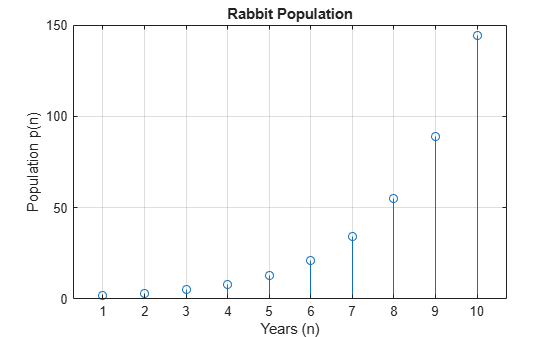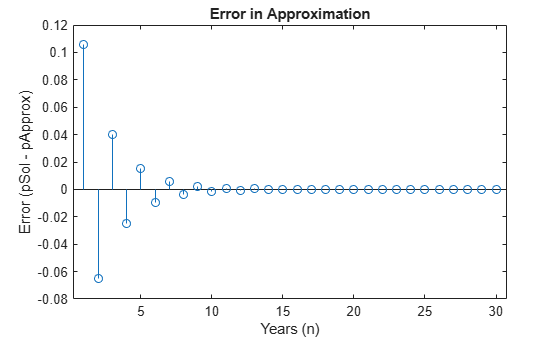# Solve Difference Equations Using Z-Transform

Solve difference equations by using Z-transforms in Symbolic Math Toolbox™ with this workflow. For simple examples on the Z-transform, see `ztrans` and `iztrans`.

### Definition: Z-transform

The Z-transform of a function f(n) is defined as

`$\mathit{F}\left(\mathit{z}\right)=\sum _{\mathit{n}=0}^{\infty }\frac{\mathit{f}\left(\mathit{n}\right)}{{\mathit{z}}^{\mathit{n}}}.$`

### Concept: Using Symbolic Workflows

Symbolic workflows keep calculations in the natural symbolic form instead of numeric form. This approach helps you understand the properties of your solution and use exact symbolic values. You substitute numbers in place of symbolic variables only when you require a numeric result or you cannot continue symbolically. For details, see Choose Numeric or Symbolic Arithmetic. Typically, the steps are:

1. Declare equations.

2. Solve equations.

3. Substitute values.

4. Plot results.

5. Analyze results.

### Workflow: Solve "Rabbit Growth" Problem Using Z-Transform

#### Declare Equations

You can use the Z-transform to solve difference equations, such as the well-known "Rabbit Growth" problem. If a pair of rabbits matures in one year, and then produces another pair of rabbits every year, the rabbit population $p\left(n\right)$ at year $n$ is described by this difference equation.

`$p\left(n+2\right)=p\left(n+1\right)+p\left(n\right)$`

Declare the equation as an expression assuming the right side is `0`. Because `n` represents years, assume that `n` is a positive integer. This assumption simplifies the results.

```syms p(n) z assume(n>=0 & in(n,"integer")) f = p(n+2) - p(n+1) - p(n)```
`f = $p\left(n+2\right)-p\left(n+1\right)-p\left(n\right)$`

#### Solve Equations

Find the Z-transform of the equation.

`fZT = ztrans(f,n,z)`
`fZT = $z p\left(0\right)-z \mathrm{ztrans}\left(p\left(n\right),n,z\right)-z p\left(1\right)+{z}^{2} \mathrm{ztrans}\left(p\left(n\right),n,z\right)-{z}^{2} p\left(0\right)-\mathrm{ztrans}\left(p\left(n\right),n,z\right)$`

The function `solve` solves only for symbolic variables. Therefore, to use `solve`, first substitute `ztrans(p(n),n,z)` with the variables `pZT`.

```syms pZT fZT = subs(fZT,ztrans(p(n),n,z),pZT)```
`fZT = $z p\left(0\right)-\mathrm{pZT}-z p\left(1\right)-\mathrm{pZT} z-{z}^{2} p\left(0\right)+\mathrm{pZT} {z}^{2}$`

Solve for `pZT`.

`pZT = solve(fZT,pZT)`
```pZT =  $-\frac{z p\left(1\right)-z p\left(0\right)+{z}^{2} p\left(0\right)}{-{z}^{2}+z+1}$```

Calculate $p\left(n\right)$ by computing the inverse Z-transform of `pZT`. Simplify the result.

```pSol = iztrans(pZT,z,n); pSol = simplify(pSol)```
```pSol =  ```

#### Substitute Values

To plot the result, first substitute the values of the initial conditions. Let `p(0)` and `p(1)` be `1` and `2`, respectively.

`pSol = subs(pSol,[p(0) p(1)],[1 2])`
```pSol =  $4 {\left(-1\right)}^{n/2} \mathrm{cos}\left(n \left(\frac{\pi }{2}+\mathrm{asin}\left(\frac{1}{2} \mathrm{i}\right)\right)\right)-\frac{3 {2}^{2-n} \sqrt{5} {\left(\sqrt{5}+1\right)}^{n-1}}{10}+\frac{3 {2}^{1-n} \sqrt{5} {\left(1-\sqrt{5}\right)}^{n-1}}{5}$```

#### Plot Results

Show the growth in rabbit population over time by plotting `pSol`.

```nValues = 1:10; pSolValues = subs(pSol,n,nValues); pSolValues = double(pSolValues); pSolValues = real(pSolValues); stem(nValues,pSolValues) title("Rabbit Population") xlabel("Years (n)") ylabel("Population p(n)") grid on```#### Analyze Results

The plot shows that the solution appears to increase exponentially. However, because the solution `pSol` contains many terms, finding the terms that produce this behavior requires analysis.

Because all the functions in `pSol` can be expressed in terms of `exp`, rewrite `pSol` to `exp`. Simplify the result by using `simplify` with `80` additional simplification steps. Now, you can analyze `pSol`.

```pSol = rewrite(pSol,"exp"); pSol = simplify(pSol,"Steps",80)```
```pSol =  $\frac{2 {2}^{n}}{{\left(\sqrt{5}-1\right)}^{n}}+\frac{2 {\left(1-\sqrt{5}\right)}^{n}}{{2}^{n}}-\frac{6 \sqrt{5} {\left(\sqrt{5}+1\right)}^{n}}{5 {2}^{n} \left(\sqrt{5}+1\right)}-\frac{6 \sqrt{5} {\left(1-\sqrt{5}\right)}^{n}}{5 {2}^{n} \left(\sqrt{5}-1\right)}$```

Visually inspect `pSol`. Notice that `pSol` is a sum of terms. Each term is a ratio that can increase or decrease as `n` increases. For each term, you can confirm this hypothesis in several ways:

• Check if the limit at `n = Inf` goes to `0` or `Inf` by using `limit`.

• Plot the term for increasing `n` and check behavior.

• Calculate the value at a large value of `n`.

For simplicity, use the third approach. Calculate the terms at `n = 100`, and then verify the approach. First, find the individual terms by using `children`, substitute for `n`, and convert to double.

```pSolTerms = children(pSol); pSolTerms = [pSolTerms{:}]; pSolTermsDbl = subs(pSolTerms,n,100); pSolTermsDbl = double(pSolTermsDbl)```
```pSolTermsDbl = 1×4 1021 × 1.5841 0.0000 -0.6568 -0.0000 ```

The result shows that some terms are `0` while other terms have a large magnitude. Hypothesize that the large-magnitude terms produce the exponential behavior. Approximate `pSol` with these terms.

```idx = abs(pSolTermsDbl)>1; % use arbitrary cutoff pApprox = pSolTerms(idx); pApprox = sum(pApprox)```
```pApprox =  $\frac{2 {2}^{n}}{{\left(\sqrt{5}-1\right)}^{n}}-\frac{6 \sqrt{5} {\left(\sqrt{5}+1\right)}^{n}}{5 {2}^{n} \left(\sqrt{5}+1\right)}$```

Verify the hypothesis by plotting the approximation error between `pSol` and `pApprox`. As expected, the error goes to `0` as `n` increases. This result demonstrates how symbolic calculations help you analyze your problem.

```Perror = pSol - pApprox; nValues = 1:30; Perror = subs(Perror,n,nValues); stem(nValues,Perror) xlabel("Years (n)") ylabel("Error (pSol - pApprox)") title("Error in Approximation")```### References

 Andrews, L.C., Shivamoggi, B.K., Integral Transforms for Engineers and Applied Mathematicians, Macmillan Publishing Company, New York, 1986

 Crandall, R.E., Projects in Scientific Computation, Springer-Verlag Publishers, New York, 1994

 Strang, G., Introduction to Applied Mathematics, Wellesley-Cambridge Press, Wellesley, MA, 1986